# RD Sharma Solutions Ex-6.4, (Part -1), Factorization Of Polynomials, Class 9, Maths Class 9 Notes | EduRev

## Class 9 : RD Sharma Solutions Ex-6.4, (Part -1), Factorization Of Polynomials, Class 9, Maths Class 9 Notes | EduRev

The document RD Sharma Solutions Ex-6.4, (Part -1), Factorization Of Polynomials, Class 9, Maths Class 9 Notes | EduRev is a part of the Class 9 Course RD Sharma Solutions for Class 9 Mathematics.
All you need of Class 9 at this link: Class 9

In each of the following, use factor theorem to find whether polynomial g(x) is a factor of polynomial f(x) , or not : (1 – 7)

Q1. f(x) = x3–6x2+11x–6,g(x) = x–3

Sol :

Here , f(x) = x3–6x2+11x–6

g(x) = x – 3

To prove that g(x) is the factor of f(x) ,

we should show ⇒ f(3) = 0

here , x – 3 = 0

⇒ x = 3

Substitute the value of x in f(x)

f(3) = 33–6∗(3)2+11(3)–6

= 27 – (6*9) + 33 – 6

= 27 – 54 + 33 – 6

= 60 – 60

= 0

Since, the result is 0 g(x) is the factor of f(x)

Q2. f(x) = 3x4+17x3+9x2–7x–10,g(x) = x+5

Sol :

Here , f(x) = 3x4+17x3+9x2–7x–10

g(x) = x + 5

To prove that g(x) is the factor of f(x) ,

we should show ⇒ f(-5) = 0

here , x + 5 = 0

⇒ x = -5

Substitute the value of x in f(x)

f(−5)=3(−5)4+17(−5)3+9(−5)2–7(−5)–10

= (3 * 625) + (12 * (-125)) +(9*25) + 35 – 10

= 1875 – 2125 + 225 + 35 – 10

= 2135 – 2135

= 0

Since, the result is 0 g(x) is the factor of f(x)

Q3. f(x) = x5+3x4–x3–3x2+5x+15,g(x) = x+3

Sol :

Here , f(x) = x5+3x4–x3–3x2+5x+15

g(x) = x + 3

To prove that g(x) is the factor of f(x) ,

we should show ⇒ f(-3) = 0

here , x + 3 = 0

⇒ x = -3

Substitute the value of x in f(x)

f(−3)=(−3)5+3(−3)4–(−3)3–3(−3)2+5(−3)+15

= -243 + 243 + 27 – 27 – 15 + 15

= 0

Since, the result is 0 g(x) is the factor of f(x)

Q4. f(x) = x3–6x2–19x+84,g(x)=x–7

Sol :

Here , f(x) = x3–6x2–19x+84

g(x) = x – 7

To prove that g(x) is the factor of f(x) ,

we should show ⇒ f(7) = 0

here , x – 7 = 0

⇒ x = 7

Substitute the value of x in f(x)

f(7) = 73–6(7)2–19(7)+84

= 343 – (6*49) – (19*7) + 84

= 342 – 294 – 133 + 84

= 427 – 427

= 0

Since, the result is 0 g(x) is the factor of f(x)

Q5. f(x) = 3x3+x2–20x+12,g(x) = 3x–2

Sol :

Here , f(x)=3x3+x2–20x+12

g(x) = 3x – 2

To prove that g(x) is the factor of f(x) ,

we should show  ⇒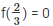here , 3x – 2 = 0

⇒ 3x = 2

⇒ x = 2/3

Substitute the value of x in f(x)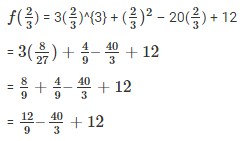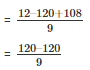= 0

Since, the result is 0 g(x) is the factor of f(x)

Q6. f(x) = 2x3–9x2+x+13,g(x) = 3–2x

Sol :

Here , f(x) = 2x3–9x2+x+13

g(x) = 3 – 2x

To prove that g(x) is the factor of f(x) ,

To prove that g(x) is the factor of f(x) ,

we should show ⇒ f(3/2) = 0

here , 3 – 2x = 0

⇒ -2x = -3

⇒ 2x = 3

⇒ x = 3/2

Substitute the value of x in f(x)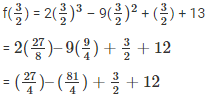Taking L.C.M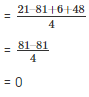Since, the result is 0 g(x) is the factor of f(x)

Q7. f(x) = x3–6x2+11x–6,g(x) = x2–3x+2

Sol :

Here , f(x) = x3–6x2+11x–6

g(x) = x2–3x+2

First we need to find the factors of x2–3x+2

⇒ x2–2x–x+2

⇒ x(x – 2) -1(x – 2)

⇒ (x – 1) and (x – 2) are the factors

To prove that g(x) is the factor of f(x) ,

The results of f(1) and f(2) should be zero

Let , x – 1 = 0

x = 1

substitute the value of x in f(x)

f(1) = 13–6(1)2+11(1)–6

= 1 – 6 + 11 – 6

= 12 – 12

= 0

Let , x – 2 = 0

x = 2

substitute the value of x in f(x)

f(2) = 23–6(2)2+11(2)–6

= 8 – (6 * 4) + 22 – 6

= 8 – 24 + 22 – 6

= 30 – 30

= 0

Since, the results are 0 g(x) is the factor of f(x)

Q8. Show that (x – 2) , (x + 3) and (x – 4) are the factors of x3–3x2–10x+24

Sol :

Here , f(x) = x3–3x2–10x+24

The factors given are (x – 2) , (x + 3) and (x – 4)

To prove that g(x) is the factor of f(x) ,

The results of f(2) , f(-3) and f(4) should be zero

Let , x – 2 = 0

⇒ x = 2

Substitute the value of x in f(x)

f(2) = 23–3(2)2–10(2)+24

= 8 – (3 * 4) – 20 + 24

= 8 – 12 – 20 + 24

= 32 – 32

= 0

Let , x + 3 = 0

⇒ x = -3

Substitute the value of x in f(x)

f(-3) = (−3)3–3(−3)2–10(−3)+24

= -27 – 3(9) + 30 + 24

= -27 – 27 + 30 + 24

= 54 – 54

= 0

Let , x – 4 = 0

⇒ x = 4

Substitute the value of x in f(x)

f(4) = (4)3–3(4)2–10(4)+24

= 64 – (3*16) – 40 + 24

= 64 – 48 – 40 + 24

= 84 – 84

= 0

Since, the results are 0 g(x) is the factor of f(x)

Q9. Show that (x + 4) , (x – 3) and (x – 7) are the factors of x3–6x2–19x+84

Sol :

Here , f(x) = x3–6x2–19x+84

The factors given are (x + 4) , (x – 3) and (x – 7)

To prove that g(x) is the factor of f(x) ,

The results of f(-4) , f(3) and f(7) should be zero

Let, x + 4 = 0

⇒ x = -4

Substitute the value of x in f(x)

f(-4) = (−4)3–6(−4)2–19(−4)+84

= -64 – (6 * 16) – ( 19 * (-4)) + 84

= -64 – 96 + 76 + 84

= 160 – 160

= 0

Let, x – 3 = 0

⇒ x = 3

Substitute the value of x in f(x)

f(3) = (3)3–6(3)2–19(3)+84

= 27 – (6 * 9) – ( 19 * 3) + 84

= 27 – 54 – 57 + 84

= 111 – 111

= 0

Let, x – 7 = 0

⇒ x = 7

Substitute the value of x in f(x)

f(7) = (7)3–6(7)2–19(7)+84

= 343 – (6 * 49) – ( 19 * 7) + 84

= 343 – 294 – 133 + 84

= 427 – 427

= 0

Since, the results are 0 g(x) is the factor of f(x)

Q10. For what value of a is (x – 5) a factor of x3–3x2+ax–10

Sol :

Here, f(x) = x3–3x2+ax–10

By factor theorem

If (x – 5) is the factor of f(x) then , f(5) = 0

⇒ x – 5 = 0

⇒ x = 5

Substitute the value of x in f(x)

f(5) = 53–3(5)2+a(5)–10

= 125 – (3 * 25) + 5a – 10

= 125 – 75 + 5a – 10

= 5a + 40

Equate f(5) to zero

f(5) = 0

⇒ 5a + 40 = 0

⇒ 5a = -40

⇒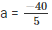= -8

When a= -8 , (x – 5) will be factor of f(x)

Q11. Find the value of a such that (x – 4) is a factor of 5x3–7x2–ax–28

Sol :

Here, f(x) = 5x3–7x2–ax–28

By factor theorem

If (x – 4) is the factor of f(x) then , f(4) = 0

⇒ x – 4 = 0

⇒ x = 4

Substitute the value of x in f(x)

f(4) = 5(4)3–7(4)2–a(4)–28

= 5(64) – 7(16) – 4a – 28

= 320 – 112 – 4a – 28

= 180 – 4

Equate f(4) to zero, to find a

f(4) = 0

⇒ 180 – 4a = 0

⇒ -4a = -180

⇒ 4a = 180

⇒ a = 180/4

⇒ a = 45

When a = 45 , (x – 4) will be factor of f(x)

Q12. Find the value of a, if (x + 2) is a factor of 4x4+2x3–3x2+8x+5a

Sol :

Here, f(x) = 4x4+2x3–3x2+8x+5a

By factor theorem

If (x + 2) is the factor of f(x) then , f(-2) = 0

⇒ x + 2 = 0

⇒ x = -2

Substitute the value of x in f(x)

f(-2) = 4(−2)4+2(−2)3–3(−2)2+8(−2)+5a

= 4(16) + 2(-8) – 3( 4) – 16 + 5a

= 64 – 16 – 12 – 16 + 5a

= 5a + 20

equate f(-2) to zero

f(-2) = 0

⇒ 5a + 20 = 0

⇒ 5a = -20

⇒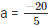⇒ a = -4

When a = -4 , (x + 2) will be factor of f(x)

Q13. Find the value of k if x – 3 is a factor of k2x3–kx2+3kx–k

Sol :

Let f(x) = k2x3–kx2+3kx–k

From factor theorem if x – 3 is the factor of f(x) then f(3) = 0

⇒ x – 3 = 0

⇒ x = 3

Substitute the value of x in f(x)

f(3) = k2(3)3–k(3)2+3k(3)–k

= 27k2–9k+9k – k

= 27k2–k

= k(27k – 1)

Equate f(3) to zero, to find k

⇒ f(3) = 0

⇒ k(27k – 1) = 0

⇒ k = 0 and 27k – 1 = 0

⇒ k = 0 and 27k = 1

⇒ k = 0 and k = 12/7

When k = 0 and 12/7 , (x – 3) will be the factor of f(x)

Offer running on EduRev: Apply code STAYHOME200 to get INR 200 off on our premium plan EduRev Infinity!

91 docs

,

,

,

,

,

,

,

,

,

,

,

,

,

,

,

,

,

,

,

,

,

,

,

,

,

,

,

,

,

,

,

,

,

;